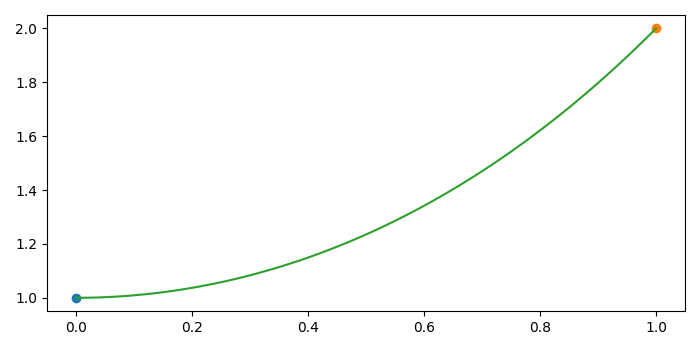# Draw a curve connecting two points instead of a straight line in matplotlib

To draw a curve connecting two points instead of a straight line in matplotlib, we can take the following steps −

• Set the figure size and adjust the padding between and around the subplots.
• Define a draw_curve() method to make a curve with a mathematical expression.
• Plot point1 and point2 data points.
• Plot x and y data points returned from the draw_curve() method.
• To display the figure, use show() method.

## Example

import matplotlib.pyplot as plt
import numpy as np

plt.rcParams["figure.figsize"] = [7.00, 3.50]
plt.rcParams["figure.autolayout"] = True

def draw_curve(p1, p2):
a = (p2 - p1) / (np.cosh(p2) - np.cosh(p1))
b = p1 - a * np.cosh(p1)
x = np.linspace(p1, p2, 100)
y = a * np.cosh(x) + b
return x, y

p1 = [0, 1]
p2 = [1, 2]
x, y = draw_curve(p1, p2)
plt.plot(p1, p1, 'o')
plt.plot(p2, p2, 'o')
plt.plot(x, y)
plt.show()

## Output

It will produce the following output